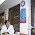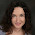## Friday, January 17, 2014

### Why the sum of all natural numbers is not negative one twelfth

This video posted by Numberphile has been making the rounds lately, supposedly proving that the sum of all natural numbers all the way to infinity is equal to negative one twelfth. The guy in the video, Tony, uses pretty basic math to derive this formula and at first glance it may not be immediately clear what is wrong with his derivation. To add to the mystery, he flashes a page from a String Theory book which quotes the formula, albeit in a very different context.

Despite a lot of controversy this video seems to be generating lately, I don't see a good explanation of what exactly is wrong with this proof among the top Google results, so I'm going to try to make it clear here.

Main points

The derivation uses "..." to denote something different in the case of computing S1 and something else in the case of S2, hence the entire derivation is invalid.

There is no interpretation of "..." which, if used consistently alongside sums of natural numbers, would lead to S = 1 + 2 + 3 + ... = -1/12.

Now, mathematical models may exist which use similar notation to the one used for integers and sums, but model phenomena other than natural numbers. Such models may abide by different rules and you may get results which don't carry over to the natural number world. This is probably what the String Theory book is doing (you can check it out at Google Books here). But these mathematical models would still have to be internally consistent in order to be useful, unlike the Numberphile framework which could be used to derive contradictory results, hence has no application other than entertainment.

Details

The first part or the proof concludes that:

S1 = 1 - 1 + 1 - 1 + 1 - 1 ... = 1/2

The argument behind this is that if the sum were cut short at any given point: if this point were odd, the sum would be 1 and if it were even, the sum would be 0. Tony goes on to say: "Do we stop at an odd or even point? We don't know, so we take the average of the two."

Note that this whole argument relies on the assumption that we do stop the series at some point. We just don't know if this point is odd or even, but not stopping at all is not an option. This is an essential distinction.

The traditional mathematical definition of infinite sums, assumes that you never stop. Since you never stop, you never stop at a point which is odd or even, hence the sum is undefined (we'd call this a divergent series).

So what Tony is really computing here, is not the infinite sum, but the expected value of a finite sum stopped at a random place. In more verbose notation we would write what Tony said as:

S1 = 1 - 1 + 1 - 1 + 1 - 1 ... (n times)
S1 = {0 when n is even; 1 when n is odd}
P(n is even) = 1/2
P(n is odd) = 1/2
E(S1) = 1/2 * 0 + 1/2 * 1 = 1/2

There would be nothing wrong with using the infinite sum notation to denote the expected value of a finite sum. In fact, the above definition is called the Cesàro sum and is a well-known mathematical concept. The problem is that having chosen the Cesàro sum as the interpretation of "...", Tony would have to stick to this definition for the remainder of the proof. But he does not do that. In fact, for the rest of the proof, he uses the same notation to mean the actual infinite sum, which never stops.

This is clear, for example, in his derivation of S2. The derivation of S2 is based on adding the series to a version of itself shifted by one, like so:

 S2 = 1 - 2 + 3 - 4 + 5 - 6 ... 2 * S2 = 1 - 2 + 3 - 4 + 5 - 6 ... + 1 - 2 + 3 - 4 + 5 ...

This is a perfectly valid operation when you are dealing with actual infinite series, because those go on forever. So even if you shift them, they can still align perfectly all the way to infinity. But it does not work under the expected value definition, since the expected value definition assumes that the series stops at some point, in which case the shifted version of S2 would have an extra term at the end which would not align with the un-shifted version.

With all this in mind, here is the video again:

1.If the series starting with +1 is S, then the series starting with -1 can be expressed both as -1 + S and as -S, and the ... in both cases mean "infinite terms follow".

if -1 + S and -S are the same and have the same sum X, then -1+X = -X, so X=1/2

1.This assumes that X is a well-defined value in the first place. If X were well-defined then your argument would show that it must be equal to 1/2. But there is no reason to assume that the sum exists - and if you think of the sum as an infinite series, X does *not* exist, because the series diverges.)

2.Excellent and Concise explanation . When we try to define something that is undefined by the rules of the game we end up with paradox like this .

3.There's nothing wrong with S1 = 1/2. If you don't like the explanation using cesaro sums you can do it equivalently by 1 - s1 = s1.

Both sides of the equation are termwise equal and with an equivalently infinite number of terms. It's a genuine equality.

You don't have to assume anything there to get S1 = 1/2. Just that infinite really means what it says.

4.You didn't disprove anything. Merely pointed out some arguments which they didn't explain in detail. The rules in this case make perfect sense and are proven by physics (see the casimir effect) they are just not intuitive to us.

5.This comment has been removed by the author.

6.This comment has been removed by the author.

7.Blah. Everyone, the author fails to address the two other derivations in the other video. One using the Reimann Zeta Function and the other simply using the summation of squares formula defined for x<1.

I highly doubt a huge proof in the mathematical community could be foiled by a single blog. If she was serious she would be publishing a paper, not writing a blog. She would get so much fame for disproving this.

8.There is no need to publish a paper, since it is a well-accepted fact that divergent series have no well-defined sum, I am not saying anything controversial here. See for example: http://mathworld.wolfram.com/DivergentSeries.html.

The problem with the derivation in the first Numberphile video is that using the same "logic" I could derive also other results. A mathematical framework which allows one to arrive at different conclusions is not very useful. I was just trying to explain where the inconsistency lies in an approachable way.

Now the Riemann zeta approach is a whole different story, since it actually does provide a consistent framework under which you get the same results no matter how you approach the problem and I am not arguing its usefulness. But this framework is not operating on natural numbers anymore, but an abstract analytic continuation of the numbers domain. Results you get in this abstract domain do not have to carry over to the natural numbers domain, so there is no conflict here. When you talk about real-world natural numbers, the divergent series sum is still infinity or simply undefined.

1.This comment has been removed by the author.

2.Hi, I saw the Riemmann zeta approach shown in this link. https://www.youtube.com/watch?v=E-d9mgo8FGk

It looks logical except that I've seen in other math sources that a sum of a geometric series is bound for IxI <1. The fellow in this video says that simply x<1, which naturally allows him later to use x= -1 in the derived formulas giving rise to the weird result of -1/12. However, if the right condition is IxI < 1 then x can only take values between -1 and 1 but not including -1 nor 1. Any thoughts about who is actually right?

9.Your argument would be more convincing if you could provide an example where you use their logic to derive an inconsistent result. Anyway, thanks for sharing your thoughts on the matter.

10.Thanks, Tom. Here is a simple example of how you can use their logic to derive an inconsistent result: Let's take the first series S1 = 1 - 1 + 1 - 1 + 1 - 1 ... = 1/2. If this is an actual infinite sum, then we can replace each (1 - 1) with (1 - 1 + 0) without changing the result (since adding zero doesn't change anything, right?) This means that S1 = 1 - 1 + 0 + 1 - 1 + 0 + 1 - 1 + 0 ... = 1/2. But if you now try to apply the "expected value" logic to the new version of S1, you conclude that if you stop the series at position 3k + 1, then the sum is 1, if you stop it at position 3k + 2, then the sum is 0, and if you stop it at position 3k, then it is also 0. Averaging these three possible outcomes gives 1/3 and 1/3 <> 1/2, hence inconsistent result.

11.Iwona, one cannot insert additional terms (yes, even zeroes) into a divergent series.

12.Yes 0+0+0+0+0+0..... = 1

13.Yes 0+0+0+0+0+0..... = 1

14.The falsehood of the notion that the sum of positive integers is -(1/12) is so incredibly, staggeringly obvious, that I have to wonder if anyone would be arguing with the OP if she weren't a woman.